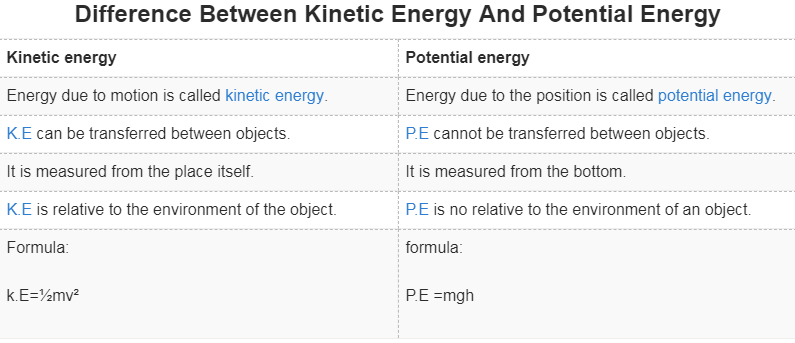Mechanics

# Difference between kinetic energy and potential energy in tabular formKinetic energy and potential energy are forms of mechanical energy.  The basic difference between kinetic energy and potential energy is that “K.E is the energy possessed due to motion and P.E is the energy possessed due to the position.”These are two different forms of energy.
Now!
If you want to learn about the difference between kinetic energy and potential energy then you are in the right place. So stay with us for a few minutes.

## Difference Between Kinetic Energy And Potential Energy

 Kinetic energy Potential energy Energy due to motion is called kinetic energy. Energy due to the position is called potential energy. K.E can be transferred between objects. P.E cannot be transferred between objects. It is measured from the place itself. It is measured from the bottom. K.E is relative to the environment of the object. P.E is no relative to the environment of an object. Formula: k.E=½mv² formula: P.E =mgh

### Kinetic energy Vs Potential energy

Related topics

External references

• https://www.diffen.com/difference/Kinetic_Energy_vs_Potential_Energy